# The Physics of FLO

Dave Tutelman -- January 2, 2008

• Why is FLO important? Because it is the most simple and direct measure of the real direction of the spine and NBP.
• Why does a "spiny" shaft wobble when plucked? Because the spring forces due to bending the shaft are not in the same direction as the bend itself.
This article explores the above questions, with illustrations, analysis, and even some math -- sorry 'bout that to those of you who are trigonometriphobes.

Here are the topics we'll cover:
• Some basics of how a shaft's stiffness varies with direction.
• Don Johnson's "shaft art", which shows exact trajectories of shaft wobble.
• A few similar phenomena: Lissajous patterns on oscilloscopes, and the Y-pendulum -- an experiment you can do.
• The equations of shaft art -- they're remarkably simple and fit easily on a spreadsheet.
• Why shafts wobble in the first place, and how much they wobble.

## The Basics

Let's start with a functional description of a golf shaft in engineers' terms, not golfers' nor clubmakers' terms.

A shaft is a spring. This is very basic, but it has a number of implication that are important to understanding FLO:
1. Since the shaft is a spring, it responds to being deformed by exerting a "restoring force". If you deflect a shaft with your finger, the shaft will exert a force on your finger. The force is called a "restoring force" because it is exerted in a direction to bring the shaft back to straight. (Well, almost. We'll see why "almost" toward the end of this article.)
2. That restoring force is proportional to (a) the amount of deflection, and (b) the stiffness of the shaft. This is true of all springs. Mathematically, engineers talk about it as F=-Kx, where F is the force, x is the amount of deflection, the minus sign is because the force is in the opposite direction from the deflection, and K is the "spring constant" of the spring; in the case of a golf shaft, K is a numerical representation of the stiffness. Yes, we all know that frequency is also a numerical representation of stiffness. K is a little different; instead of cpm, it is expressed in pounds per inch of deflection (or newtons per centimeter, or other units of "force per distance").
The shaft is not only a spring; more specifically, it is a flexible beam. Note that I didn't say, "Perfectly symmetrical flexible beam." We're talking about spine and FLO here, so we are exploring what happens when the cross-section of the beam is not symmetrical. That leads us to a few more facts that engineers know about flexible beams, even asymmetrical beams:
1.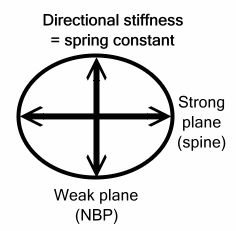If you deflect the shaft and measure the spring constant (the stiffness), then deflect it in exactly the opposite direction (that is, exactly 180º away), you will get the same spring constant. It is equally stiff in both directions.
2. If the cross-section of the beam is not perfectly symmetrical, then the beam may have a strong plane (the direction of highest spring constant) and a weak plane (the direction of lowest spring constant). Clubmakers refer to the strong plane as the spine and the weak plane as the Natural Bending Position (NBP) -- or at least they should if they are using the right measuring instruments. Repeating that as a table, for clarity:
 Strong plane Weak plane Direction of highest spring constant Direction of lowest spring constant Spine NBP

3. The strong plane and the weak plane are 90º apart.
4. Between the strong plane and the weak plane, the spring constant varies as an ellipse, as the diagram shows.
5. If the shaft were perfectly symmetrical, then the ellipse would be a circle, and the spring constant would be the same in every direction. That would be a shaft with no spine.
These rules are true no matter how asymmetrical the shaft. You could weld a steel rod to one side of the shaft, and the rules would still be true. I'm not going to explain here why this should be. But it has been well known to those studying structures for a long time. I have even found a mathematical proof of it in Timoshenko's classical mechanics book, which was written in 1930.

Before we go any further, let's state some assumptions underlying the rest of the work:
• Whatever asymmetrical stiffness the shaft has, it is the same (including its orientation) for the length of the shaft. (It turns out that this isn't a major restriction, but it's hard to show that fact so I won't bother.)
• The shaft, when flexed either for "shaft art" or by a golf swing, remains a linear spring. Almost every spring material and spring design has some level of deflection where F=-Kx fails. We assume that this doesn't happen to our shaft in normal operation.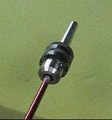One final "basic". Let's look at how a shaft oscillates when there is a spine -- how it gets from a planar up-down vibration to an out-of-plane oval. Here is a video example, using a shaft with a really substantial spine.

## Shaft Art

Sometime around 2001, something happened to me that really clarified FLO in my mind -- what it was and what it meant. Don Johnson of BTM Clubs sent me some graphs that he had made. Rather, some shafts made the graphs for him, their tips tracing out a pattern as they wobbled. They looked original and attractive, so he called them "Shaft Art", which certainly seemed appropriate.

Here's a sampling of some representative shaft art from Don's collection. (Thanks, Don.)ProLite 3-iron shaft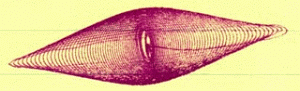ProLite 8-iron shaftAJ Tech shaftAJ Tech shaft (two different plucks)Aldila VL-1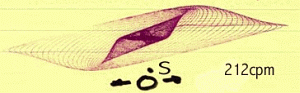Quadrax shaft

The pictures show the path of the shaft tip after it is plucked, when clamped at the butt and weighted at the tip. (Think of taking a frequency measurement, or looking for FLO.) Don made the pictures by attaching a pen to the tip of the shaft; the pen was the instrumentation pen from a Hewlett-Packard plotter. The paper was 3M Post-It notepaper, suspended by its sticky edge to minimize the friction. (Today, you'd probably use a laser pointer attached to the tip weight, and a camera to take a time exposure of the laser's trail. The resulting picture would be the same.)

My reaction the instant I saw them was, "Hey, I know what that is! I've seen it before, a long time ago."

## Oscilloscopes and Lissajous Patterns

Around 1960, when I was an undergraduate Electrical Engineering student at CCNY, I saw patterns like that in the electrical measurement lab at school. I viewed the patterns on an oscilloscope screen, and knew them as Lissajous patterns.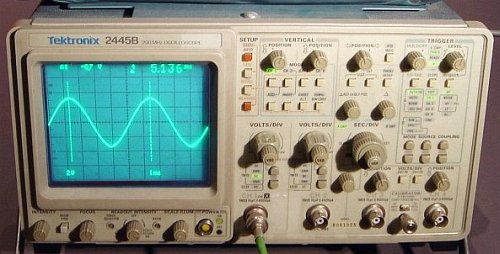This is an oscilloscope, an instrument whose purpose is to visually display electrical waveforms. Normally it is used as shown in the picture, displaying an amplitude (usually voltage) vs time. In other words, it displays a graph whose horizontal axis is time, and whose vertical axis is voltage. In the picture, the voltage is a "sine wave" -- which happens to be the same shape as a clamped shaft's motion vs time.

 But there is another mode in which you can use an oscilloscope. You can display one waveform on the horizontal axis, and another on the vertical axis.This is useful when you want to compare the frequencies of two waveforms. (Yeah, I know. Use a frequency counter. That's how it would be done today. But frequency counters were expensive and relatively rare in 1960, while every electronics lab had oscilloscopes.) Here is how it works. The example shows two sine waves of the same frequency, but "out of phase" with each other. (Out of phase means the waves do not reach their peaks at the same time.) As one wave generates the vertical deflection on the instrument's screen, the other wave generates the horizontal deflection -- instead of a time base for horizontal. As the figure shows, the result is an ellipse of some sort. If the waves are perfectly in phase, the image is a diagonal straight line, and "ellipse" with no width. If the waves are 180º out of phase, then you get a diagonal line in the other direction. And if they are 90º out of phase, the result is a circle. Mathematically, we are looking at a plot of two "parametric equations". You use parametric equations when it is hard to write an equation of x vs y, but you can write both x and y in terms of some other variable -- like time t. Both the horizontal (x) and vertical (y) waveforms are relatively simple functions of time, along with a constant phase angle φ. If φ=0, then the waves are in phase, otherwise they are out of phase.So, if the frequencies are the same, you get some kind of ellipse. But what happens if they are not the same? If they are far apart, then you get a very interesting swirly pattern, that it takes some experience to understand. But, for a clubmaker trying to understand FLO, the most interesting case is when the horizontal and vertical waveforms have almost the same frequency -- but not quite the same. When that happens, it looks like a same-frequency ellipse, except that the phase keeps changing because of the slight difference in frequency. The ellipse keeps morphing on the screen, like the animated picture here. Look familiar? Doesn't it look like a shaft with a lot of spine and no damping (so it doesn't lose amplitude), when plucked in a non-FLO direction? That isn't just a coincidence. As soon as I saw Don's shaft art, I realized that shaft wobble due to spine is mathematically the same thing as a Lissajous figure on an oscilloscope screen. And since the Lissajous pattern has remarkably simple equations defining its motion, shaft wobble is pretty simple too -- in spite of its seeming visual complexity.

## The Y-Pendulum

As a college sophomore, I thought Lissajous figures were really cool. I wanted to play with them, just for the visual experience. But I didn't get my own oscilloscope for home until my junior year, when I built one. So I had to find another way to play with them.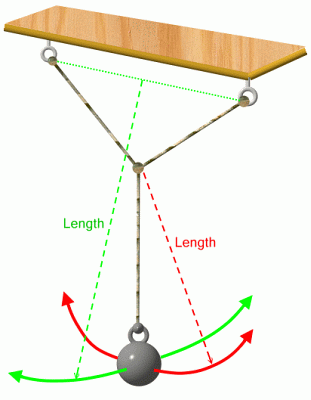I built a pendulum with two frequencies, one frequency in one direction and another frequency perpendicular to that direction. How can we do that? We make the shape of the pendulum a "Y", as in the picture. The three arms of the Y are string tied together. The pendulum is suspended at two points (instead of the usual one), and there is a weighted bob to provide the mass. We all know that the frequency of a pendulum is dependent on its length. But the Y-pendulum has two different lengths: in the plane of the Y, only the bottom portion can swing, so the length is shorter -- and thus the frequency is higher. Make one for yourself, it's certainly easy enough. Try to have the bottom arm of the Y at least 3/4 of the total height of the pendulum. Somewhere around 80-85% is ideal. Now you can play with it to confirm the following behaviors: If you release it to swing exactly in the plane of the Y (shown in red), it will swing back and forth with no wobble. If you release it to swing perpendicular to the plane of the Y (shown in green), it will swing back and forth with no wobble -- but at a slightly lower frequency (longer period) than it did in the plane of the Y. If you release it in any other direction, it will wobble. It will oval out into an ellipse, then settle into a straight line (but different from the original release). But it won't stay there. It will oval again, then return to a straight line like the original release. It behaves just like the animated oval in the previous section. It will continue this oval-straight alternation until the small friction in the string and air resistance bring it to a halt. This is exactly the behavior of a clamped shaft with a spine. Don's shaft art can be duplicated by a Y-pendulum with proper parameters. (Those parameters would include damping as well as frequency and the initial angle of release.) So what did I do with my Y-pendulum in 1960? I made it with a flashlight as the bob. I set my camera for time exposure, pointed it upward from below the pendulum, and photographed enough swings to get an interesting Lissajous pattern. The important result is... a lot of those photos looked very much like Don's shaft art. As I said, I've seen this before -- over forty years ago.

## Testing the Theory -- A Shaft-Art Spreadsheet

It's one thing to say that Don's shaft art looks like a Lissajous pattern. It's quite another to prove that it is a Lissajous pattern. Can we make that leap? Let's try.

I have come up with an Excel spreadsheet to generate Lissajous patterns. It is based on the simple parametric equations above, plus a damping factor so the oscillations decay as a real shaft's will. (You can download a copy of the spreadsheet to play with yourself.) Using it, I have duplicated enough of Don's shaft art that I am convinced that is what we are looking at. Here's a sample of shaft art and the corresponding Lissajous pattern.Shaft art - ProLite 3-ironLissajous Pattern Freq. ratio = 1.03, increment = 12.8º, decay = .0015

Impressed? I am. We'll see more below. But first let's see what underlies the spreadsheet.

As we saw before, the horizontal and vertical axes are parametric equations with time as the common parameter. Each equation is a sine wave, with the following details needing to be filled in:
• Frequency. Actually, all we need is the ratio between the horizontal and vertical frequencies. To give a feel for this, consider a driver shaft whose frequency is 250cpm. If the size of the spine is 10cpm (that is, the difference between the spine and NBP frequencies is 10cpm), then the frequency ratio is 1.04 (that's [250+10]/250).
• Phase. In order to match arbitrary shaft art, the spreadsheet supports a phase angle for both horizontal and vertical sine waves. By the way, a pure "pluck" of a shaft has both x and y starting at a phase of 90º. In order to keep the default phase at 0º (an aesthetic choice, not a mathematical necessity), we use cosine instead of sine -- because cosine is a sine wave that starts at 90º.
• Amplitude. Horizontal and vertical amplitudes must obviously be adjusted to match the aspect ratio of the shaft art. Although I have included amplitude parameters, Excel automatically scales graphs -- so changing the amplitude does not change the graph. For the graphs I show, I adjusted the amplitude by sizing the graph after it was produced.
• Decay. A shaft has internal friction, so the oscillations are damped out over time. That is, the vibrations "decay" at some rate. The way we handle this in the spreadsheet is to multiply the amplitude each sample by a number less than 1.0 in order to get the next sample. The "decay" variable is a small number indicating how rapidly the vibrations damp out. Example: if the decay variable is .0013, then the amplitude of each sample is multiplied by (1-.0013) to get the next sample. This gives the sort of exponential decay that corresponds to physical reality.
• Time window. How long do we run the simulation? How many cycles of vibration are in the shaft art we are trying to simulate? We control this in the spreadsheet by dividing the 1000 points we compute into degrees. For instance, if we want to simulate 40 cycles, then each cycle is represented by 25 points on the graph. So the step-to-step increment is 14.4º=360º/25.
• Orientation. Don's shaft art is oriented however Don had the shaft and the paper for that run. The spreadsheet is restricted to having the FLO planes horizontal and vertical. I used my favorite graphics program (ULead PhotoImpact) to rotate the graphs so they are oriented as Don's shaft art is. I didn't try to hide the rotation; that's why some of the Lissajous patterns show slanted borders in the corners.
Ultimately, the equations driving the spreadsheet look like:
x = Ax e-Dxt cos (fx t + φx)
y = Ay e-Dyt cos (fy t + φy)
where:
y = vertical distance on graph.
x = horizontal distance on graph.
t = time.
A = amplitude.
D = decay rate, which is related to the decay variable in the spreadsheet.
fy/fx = frequency ratio.
φ = phase.

These are remarkably simple equations for something as complex as shaft spine or as complex-looking as shaft art. You may not think so if you are afflicted with trigonometriphobia but, as mathematical models of the real world go, it doesn't get much easier than this.

Now let's see some more shaft art and how the spreadsheet duplicates it.

 Shaft Art Lissajous PatternProLite 8-iron shaft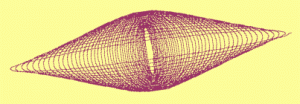Freq. ratio = 1.01, increment = 16º, decay = .0018, phasey = 6ºAJ Tech shaft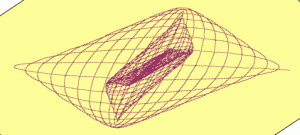Freq. ratio = 0.955, increment = 13º, decay = .0035,.0015AJ Tech shaft (two different plucks)Same as above, but two plucks superimposed, one near spine and one near NBPAldila VL-1Freq. ratio = 1.045, increment = 18º, decay = .0018,.0016Quadrax shaft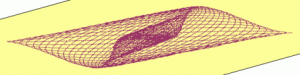Freq. ratio = 1.025, increment = 16º, decay = .002

This is a remarkable match, given such a simple mathematical model and such intricate real-world data.

## Whys and Wherefores of Shaft Wobble

### But first, a few words about science...

What we have here is, as a scientist would say, a theory that passes the data test. Before we continue investigating what we can learn from this theory, I'd like to put the word "theory" into proper context.

The two most important concepts in science are theory and law. These words have very specific meanings in a scientific context, so perhaps it's unfortunate that they also have meaning in everyday context. Here's what a scientist means:
• A law of science is a reliable, repeatable observation. It is a generalization about observed data. Example: "The sun rises and sets once for each 24-hour period," is a law. We observe that to be true over and over again. We have never observed it to be false, so it's a very solidly entrenched law.
• A theory is an explanation for an observed law or, even better, for a set of related laws. Example: Let's look at theories for the law we just mentioned. From the beginning of science up until a few hundred years ago, the prevailing theory to explain the sun's rising and setting was, "The sun revolves in an orbit around the earth." But, as more obervations were made -- many by astronomers in the era of Copernicus and Galileo -- it became clear that a different explanation better fit all the data, not just the sun's rising and setting: "The earth rotates on its axis, and causes the sun to appear to move." That theory supplanted the old theory, and remains the explanation to this day.
That is the scientific method in a nutshell! There's a lot of additional detail you need to know to practice, to understand, or to criticize scientific work, but first and foremost you have to understand this relation between theory and law. Laws are directly observed phenomena; theories are explanations of laws. Where competing theories exist to explain the same laws, the "correct" theory is selected on the basis of simplicity, consistency with more laws, and its ability to correctly predict observations that have not yet been made but can be tried.

So the next time you hear someone say, "Well, that's just a theory!" to put down an idea, remember what "theory" really means. Any scientific explanation is "just a theory"... because that's what a theory is. But some theories have stood the test of data, and are accepted by science as fact -- at least until a better theory comes along. And "better" means simpler, more consistent with observed data, and better able to predict new laws.

### Now back to our regular programming...

How does this apply to FLO and shaft art?

I have postulated a theory about the behavior of unsymmetrical golf shafts:
Unsymmetrical golf shafts behave as a pair of springs at right angles to each other, one on the strong axis of the shaft and the other on the weak axis.

Don's shaft art is data. Collectively, we can think of it as a law of science, a set of data that any of us can duplicate.

My theory is sufficiently simple that we can mathematically model the vibrating shafts. I have done so. The mathematical model is able to create the same shaft art that Don observed. So -- at least for the data we have on hand -- the theory appears to be valid. Let's treat it as valid, and see what it teaches us about shaft behavior.

Here are two important lessons if the theory is correct:
1. FLO is a reliable way to determine the strong and weak planes of a shaft.
2. If a shaft is bent in a plane other than the strong or weak plane, then the restoring force of the spring will not be in the direction of the bend.
Let's look at these in more detail...

### Finding spines

FLO is a reliable way to determine the strong and weak planes of a shaft.

How do we know this is true?

Because it follows from the theory. If the shaft behaves like two independent springs at right angles, then the vibration is the sum of what the two springs would produce independently. As we saw from the equations, that motion consists two sine waves. And the result cannot be a straight line (FLO) unless one of the following is true:
• The frequencies are the same. (In other words, the "strong" and "weak" planes have the same spring constant, so the shaft has no spine.)
• The oscillation is exactly in the strong plane (the spine plane). This is true because the weak plane spring -- with a different frequency -- is never stretched, so it never contributes to the motion.
• The oscillation is exactly in the weak plane (the NBP plane). Same reasoning.
If you don't want to deal with the math, then play with a Y-pendulum. We have shown that its motion has the same physical model as a vibrating shaft. And it only swings in a straight line (a) in the plane of the "Y", (b) perpendicular to the "Y", or (c) if the pendulum is a single string (no "Y", same period in all directions).
Why is this important?

Because conventional, bearing-based spine finders are not a reliable way to find a spine. They are thrown off by any residual bend in the shaft. If the shaft is very straight and has a lot of spine, then they will give the right answer. But, for most shafts, the residual bend is enough to partially mask a modest spine, and the spine-finder gives the wrong direction.

Now you know a way to find the spine reliably. Just find the planes of FLO. They will be the spine and the NBP.

If you have a frequency meter setup in your shop, you already have the equipment. The higher-frequency FLO plane is the spine, and the lower frequency the NBP.

Even if you don't have a frequency meter, a solid clamp for the shaft butt will do. (It must be solid enough so that neither the clamp nor the bench on which it is mounted vibrate along with the shaft.) Now you can find the two FLO planes. Distinguishing which of these planes is the strong plane and which is the weak one can sometimes be deduced with the aid of the bearing-based spine finder.

### Out-of-plane spring force

If a shaft is bent in a plane other than the strong or weak plane, then the restoring force of the spring will not be in the direction of the bend.

When you deflect a spring, the restoring force is generally in exactly the opposite direction to the deflection. So, when you bend a shaft at the tip, the restoring force should be in exactly the opposite direction to the bend. Right? Well almost. Without spines, the answer would be correct. But now we have a mathematical model for the spine; let's use it to see what the restoring force looks like.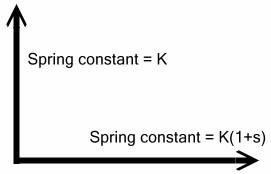First let's specify the model of the shaft. It consists of two springs, one on the weak axis (or NBP) and the other on the strong axis (or spine). In the picture, the NBP is vertical and the spine is horizontal.

Each spring has its own spring constant. Usually the spring constants would be called K1 and K2, or maybe Kx and Ky. But it turns out that the ratio of the spring constants is what we're really interested in, and that ratio is very close to one in the case of shaft spine. So let's refer to the spring constants as K for the NBP and K(1+s) for the spine.Now what happens when we bend the shaft? That depends on which direction we bend it.
• If we bend it vertically, then there is a simple restoring force -Kx1
• If we bend it horizontally, there there is a simple restoring force -K(1+s)x2
• If we bend it in any other direction, then both springs get into the action. We need to analyze that case more carefully.
Suppose we bend the shaft as an angle A to the NBP. Then the amount of bend on each axis is x1 and x2, where
 tan(A) = x2 x1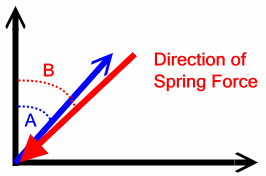How about the angle of the restoring force? It will be the resultant of two spring forces at right angles to one another.
• The vertical force is -Kx1
• The horizontal force is -K(1+s)x2
The resultant force B (shown in red in the diagram) is not necessarily at the same angle as the bend angle A. The formula for B is:
 tan(B) = -K(1+s)x2 -Kx1 =  (1+s) x2 x1So the force (B) is always a little closer to the spine than the bend itself (A). The difference angle (B-A), the angle between the bend and the spring force that results, can be computed without too much trouble:
 tan(B-A)  = s tan(A) 1 + (1+s) tan2(A)

That is just a function of s and the angle the bend is off from the NBP. Here is a plot of that function for s=.04, .08, and .12. For a shaft with a 250cpm frequency at the NBP, that corresponds to spines of 5cpm, 10cpm, and 15cpm.

### Conclusions about wobble

What does this mean in practical terms? Here are a few interesting conclusions:
1. A swing that generates only in-plane bending can still create forces that throw the clubhead out-of-plane. That's because, if the bend is not in a FLO plane, the force is not in the same direction as the bend.
2. If out-of-plane forces are the thing that causes a misaligned shaft to result in a bad club, then the size of the spine matters. Look at the graph. For a 5cpm spine, the difference angle never gets bigger than 1.12º. For a 15cpm spine, the difference angle exceeds 1.12º if the shaft bend is more than 8º from one of the FLO planes. So a 15cpm spine, if not aligned "properly" (whatever that means) to within 8º, is at least as bad as a 5cpm spine that hasn't been aligned at all -- and probably quite a bit worse.
Early tests by SST PURE in the 1990s determined that misaligned shafts resulted in a bigger variation in the impact spot on the clubface. If that is the main problem with misaligned shafts, then the out-of-plane force is probably a big part of that problem. So, if those early tests really reflect the need for spining, you have just learned why in this article. A misaligned shaft will result in out-of-plane forces that cause the clubhead to move out-of-plane during the downswing.

But we don't know for sure that this is the problem with spines. There are quite a few theories about what causes misaligned shafts to result in bad clubs. So not all the returns are in yet. But one thing at we do know: FLO is one of the most reliable ways to find the spine of a shaft.

### Notes:

1. FLO is short for Flat Line Oscillation. It refers to the way a clamped shaft vibrates. If you clamp the butt of a shaft and put a weight (or a clubhead) at the tip, you will get some pattern to the vibration. Either the tip will oscillate back and forth in a straight line (FLO), or it will wobble out of plane (non-FLO).
2. S. Timoshenko, "Strength of Materials - Part I", D. Van Nostrand, 1930, Appendices, esp. App V.
3. I have a soft spot in my heart for the H-P flatbed plotter. Back in 1970, it was involved in my first foray into mixing computers, technology, and sports. I was sailing competitively at the time, in the Albacore one-design sailboat. I used a computer to determine the cross-section of the optimum centerboard, and made the centerboard it specified. The link between numbers and reality was a set of templates, printed by computer using the H-P plotter. Nobody won a major Albacore championship for the next couple of years without first borrowing those templates and building a new centerboard from them.
4. Funny story about that! In the early 1970s, I was the manager of an electronics research group in a large telecom company. In planning our annual budgets, it was always hard to convince upper management that we needed newfangled stuff. But nobody ever questioned allocating funds for a half-dozen more oscilloscopes, even though you couldn't walk through the lab without bumping into a few and without pushing other 'scopes aside. So that's how I always got my "discretionary" budget for next year. I'd budget the dollars for oscilloscopes, and then spend on what my group really needed.
5. Shaft art is certainly a visually pleasing kind of pattern. But, if you want to know just how cool Lissajous figures can be, download the spreadsheet and try larger frequency ratios than you're likely to find on the spine of a shaft. For instance, set the increment to 10º and the decay to .0015, then try:
• Frequency ratio = 3, phase = 45º
• Frequency ratio = 1.25, phase = 0º
• Frequency ratio = 4, phase = 30º
• Frequency ratio = 1.333, phase = 22.5º, decay = .0005
6. I'll take this opportunity to thank Dr. Lawrence Podell for spending a month drumming this into my head. At the time (1958), I was a freshman in the engineering school at the City College of New York, and he was a fairly junior professor there. He must have been pretty junior, because he was teaching a social studies course for engineers. Junior or not, he was my first teacher who actually met my expectations of what a college professor should be, and he gave me what is still my most comprehensive education about what science is.
7. The curve of the angle between the bend and the restoring force looks to the eye like a sine curve, specifically
(B-A) = 27 s sin(2A)
This is a pretty good approximation for small values of s. It gets a little out of hand at about s=.25, where the spring constant is 25% greater at the NBP than at the spine. That corresponds to a whopping spine of 30cpm on a 250cpm shaft. Bet you've never seen a spine that big. So feel free to use the approximate formula.